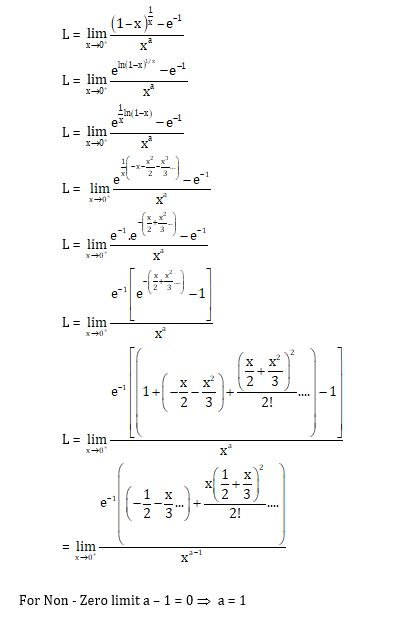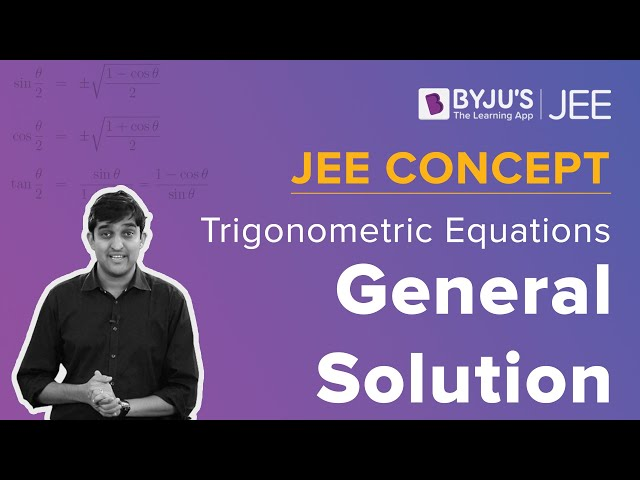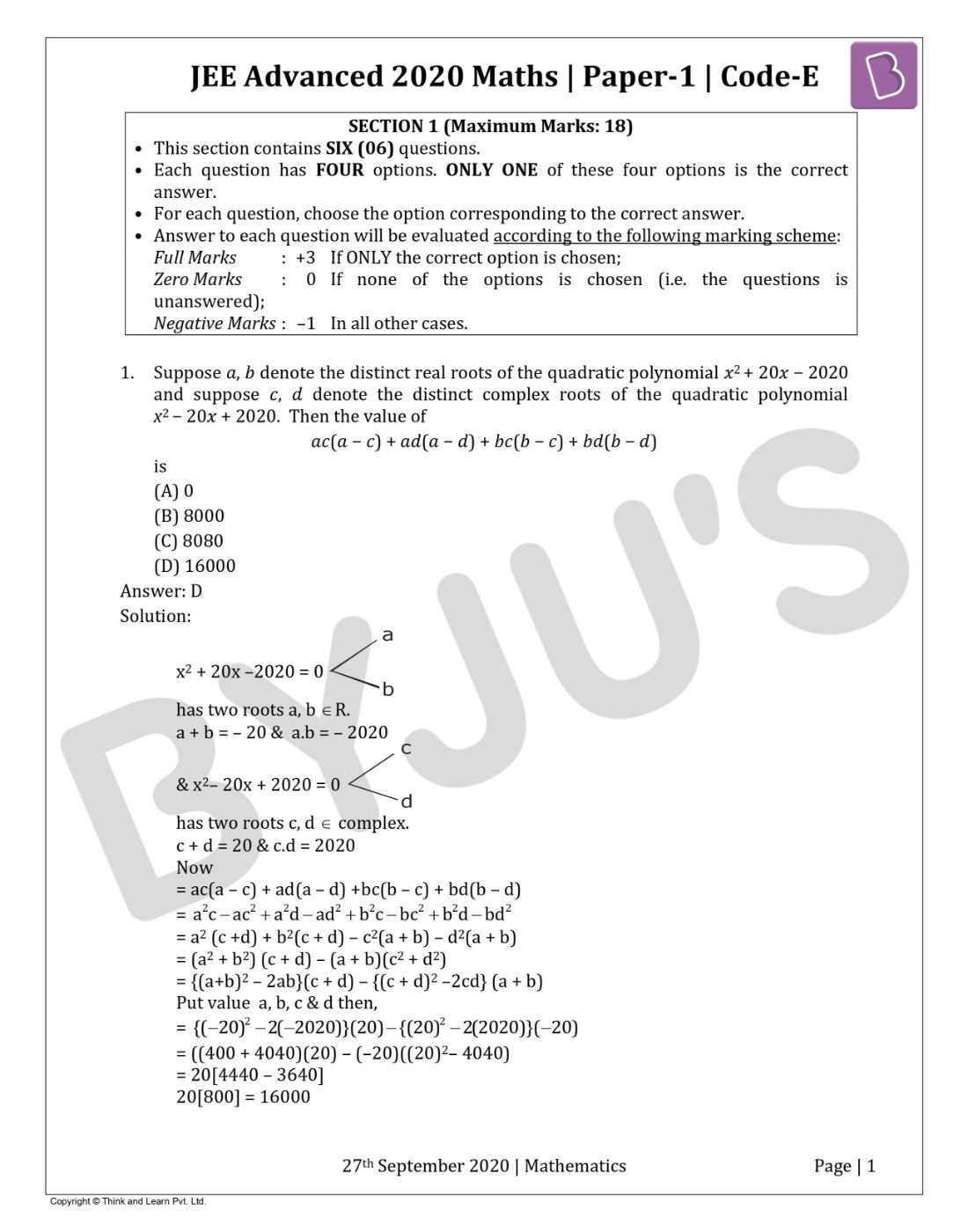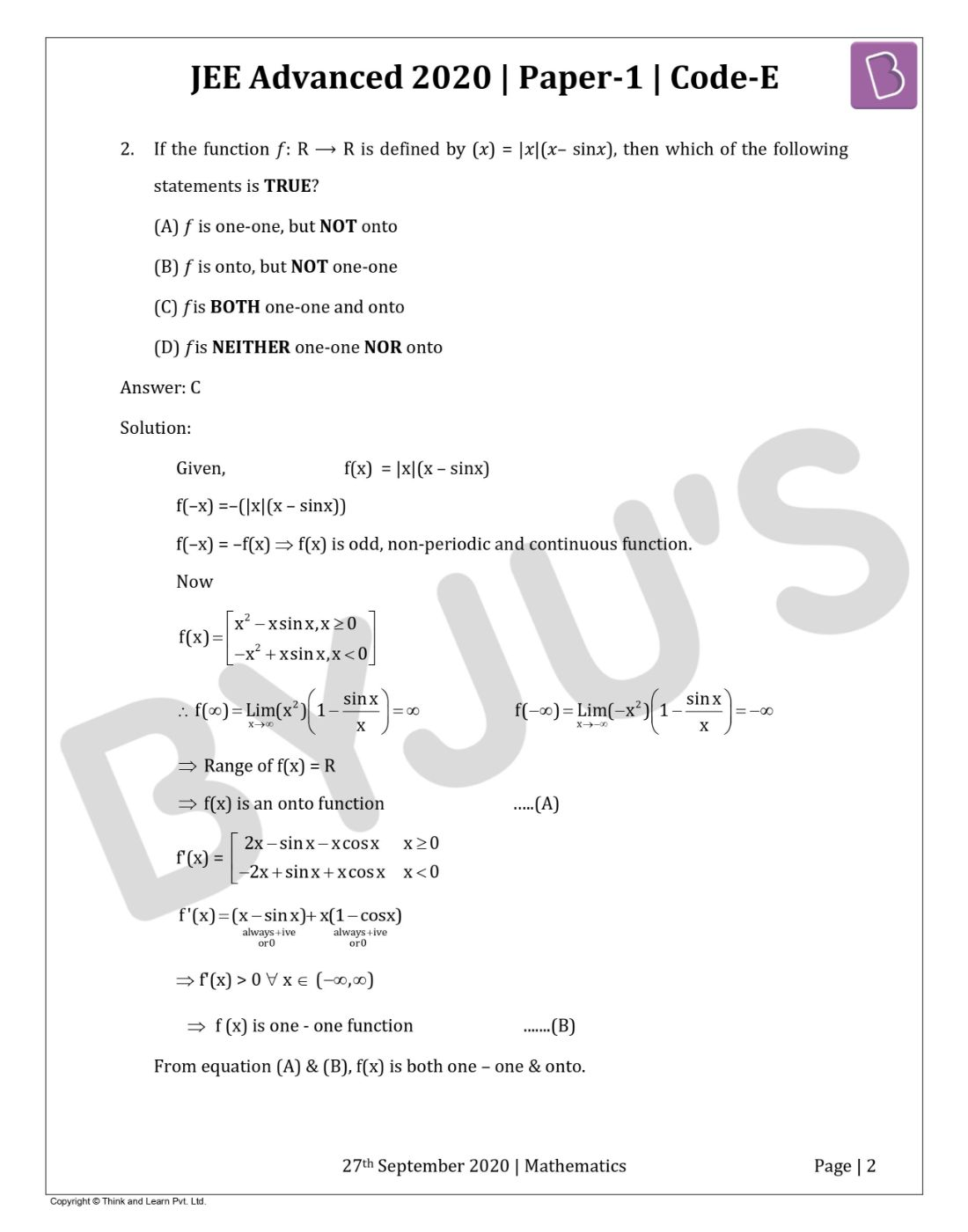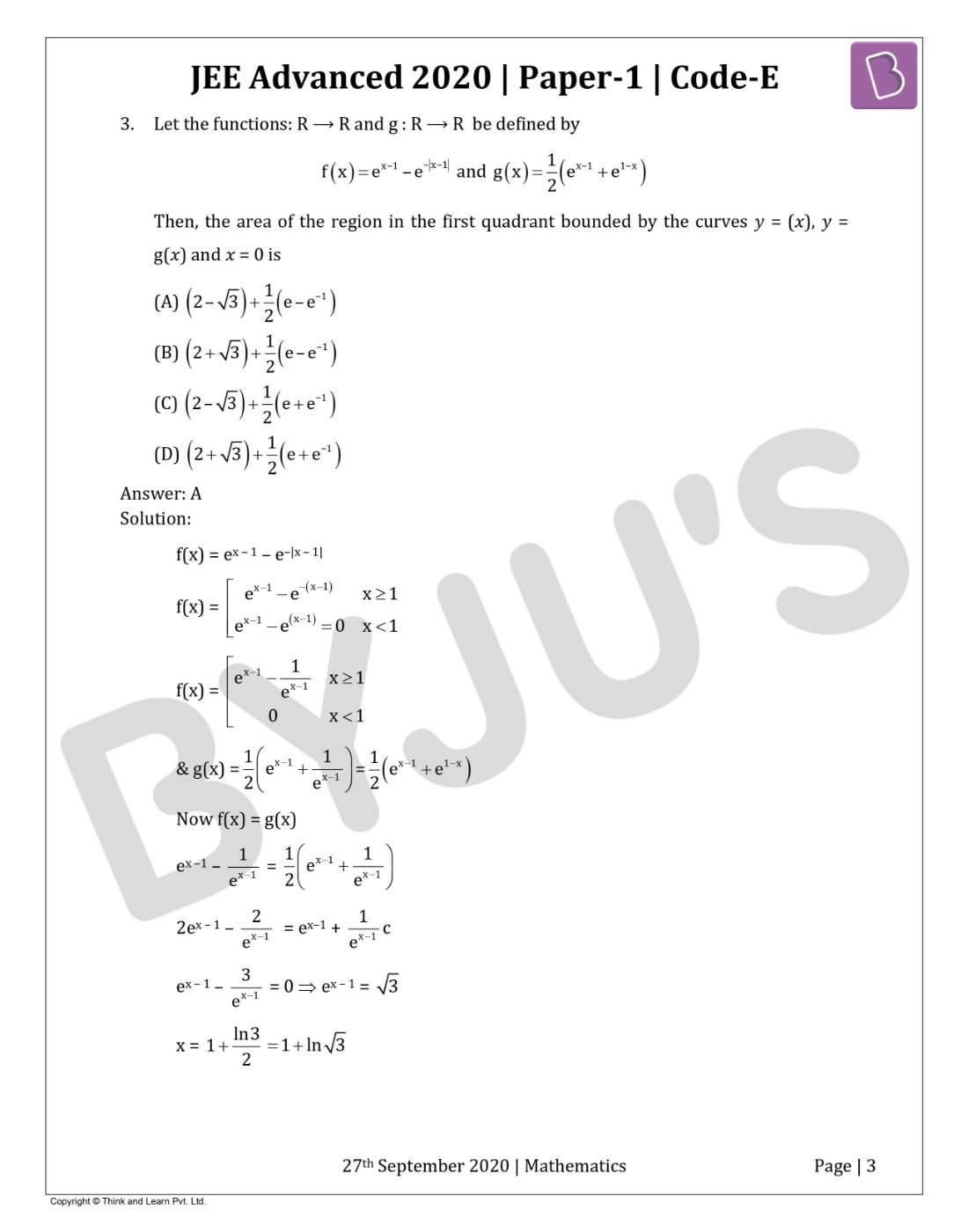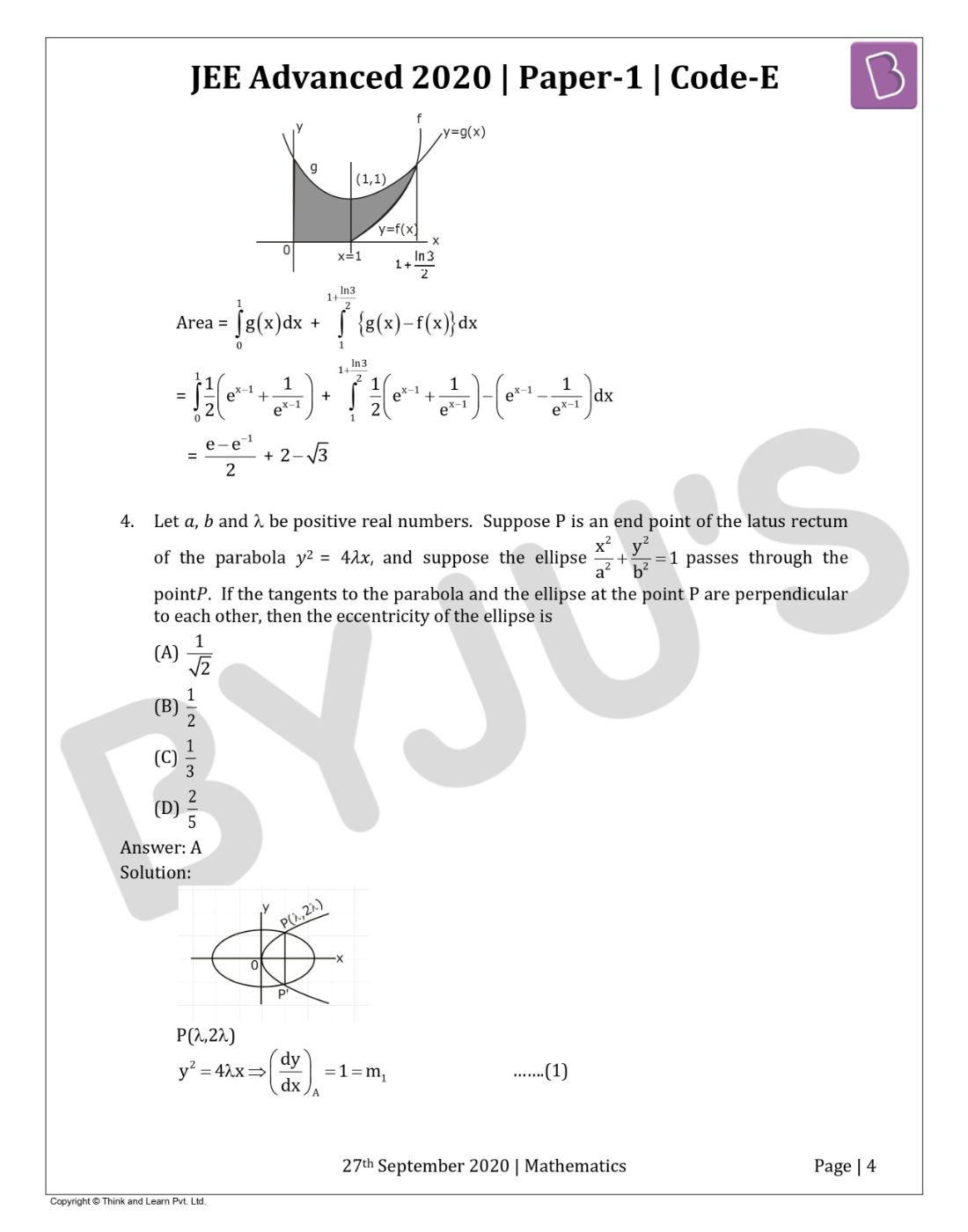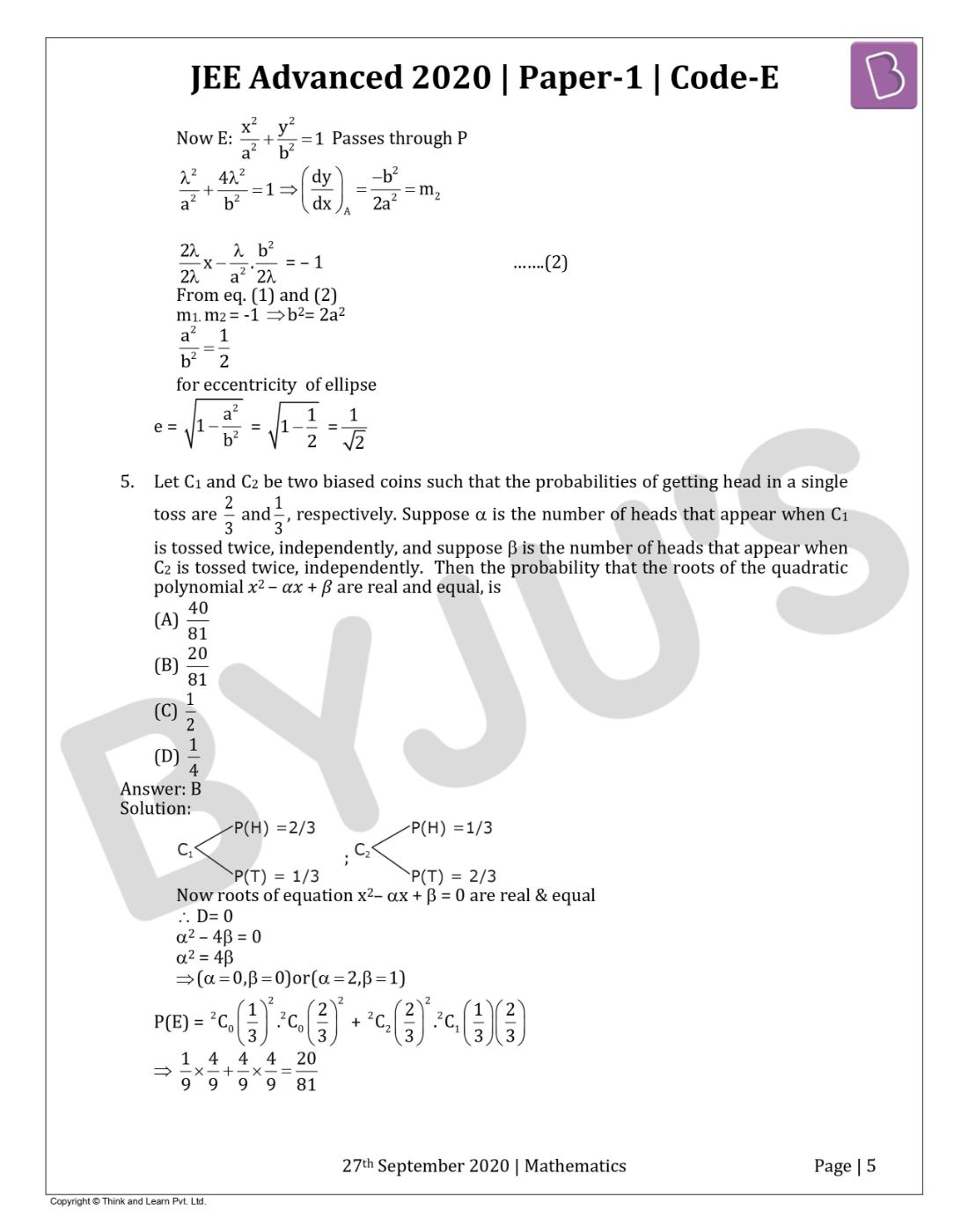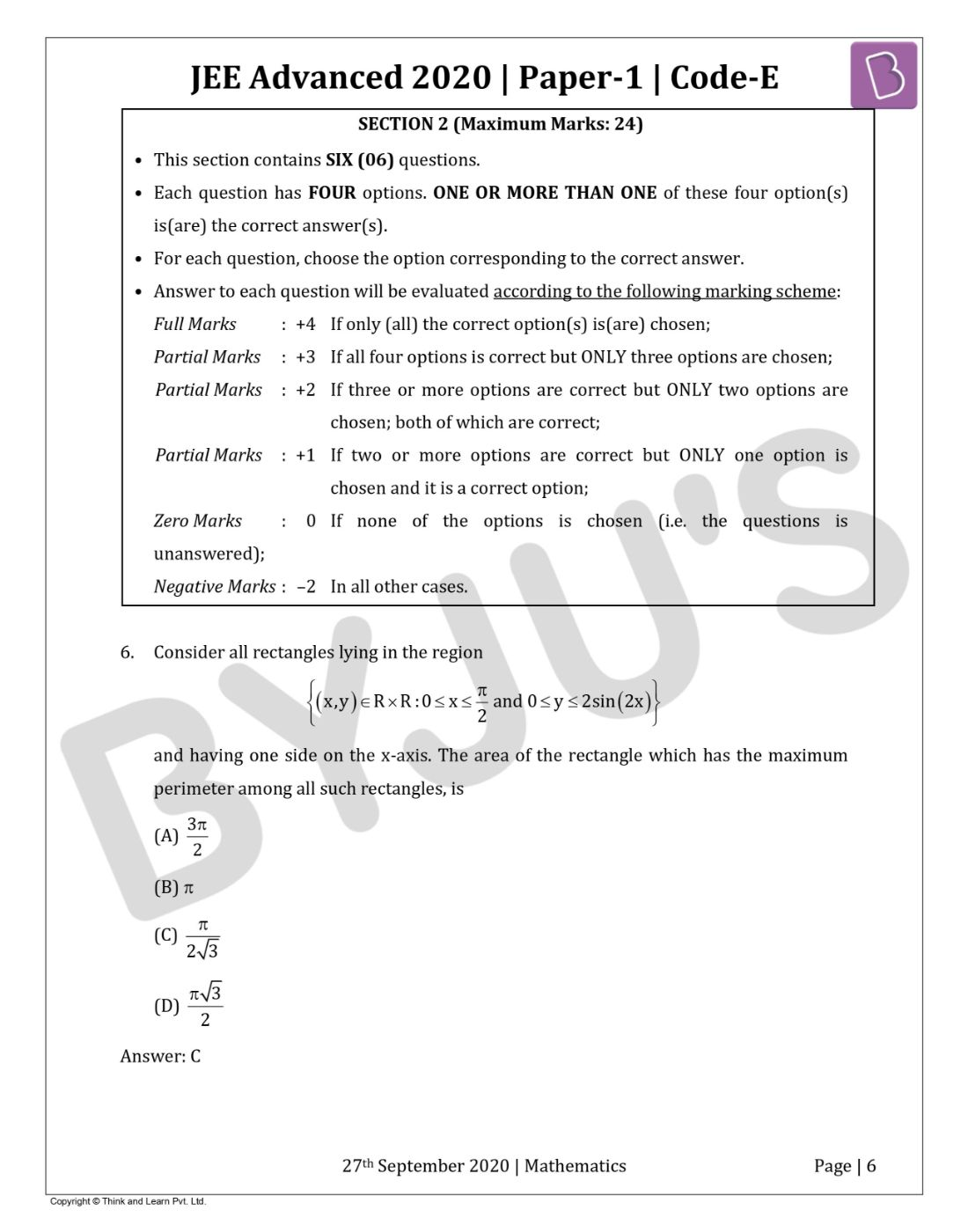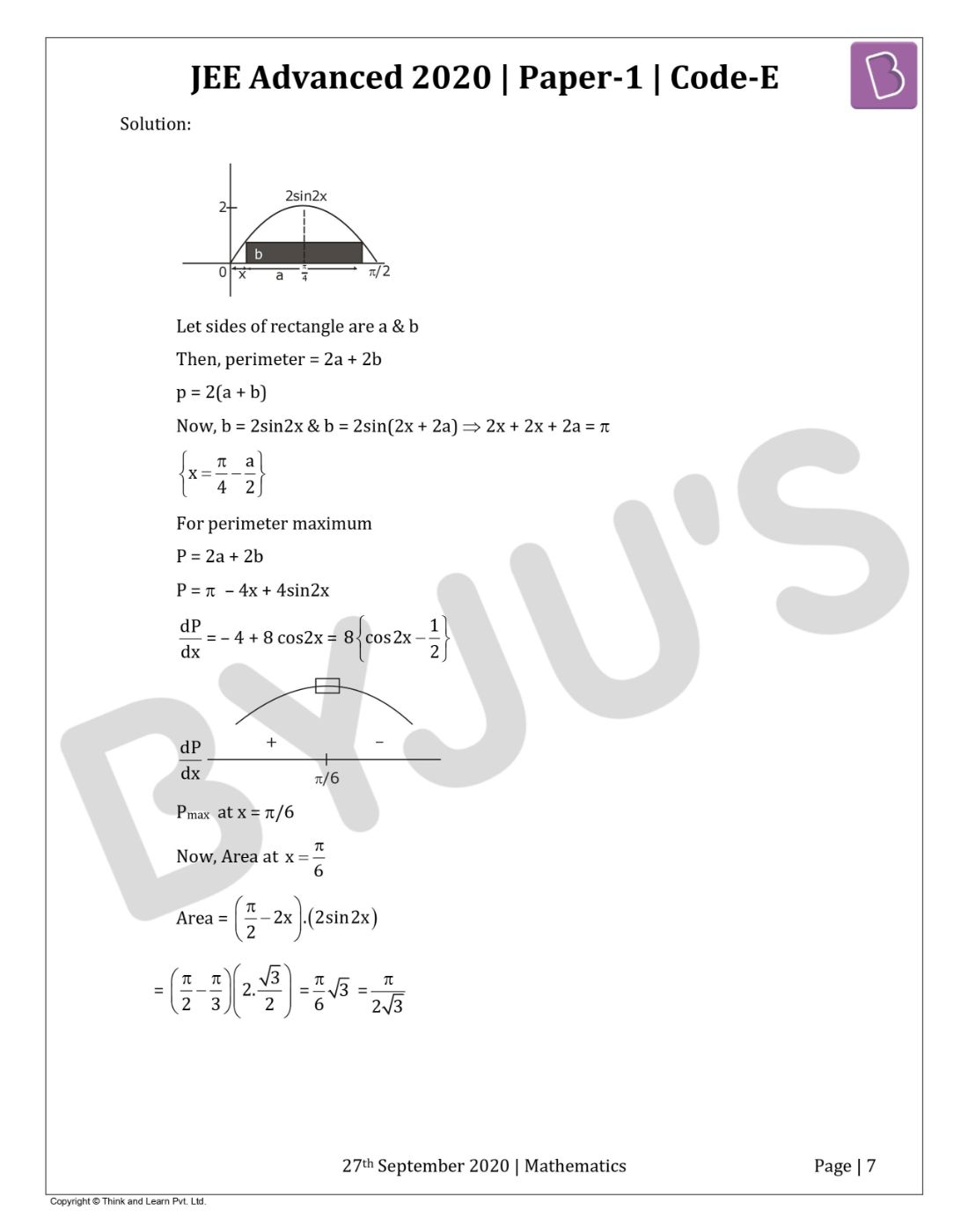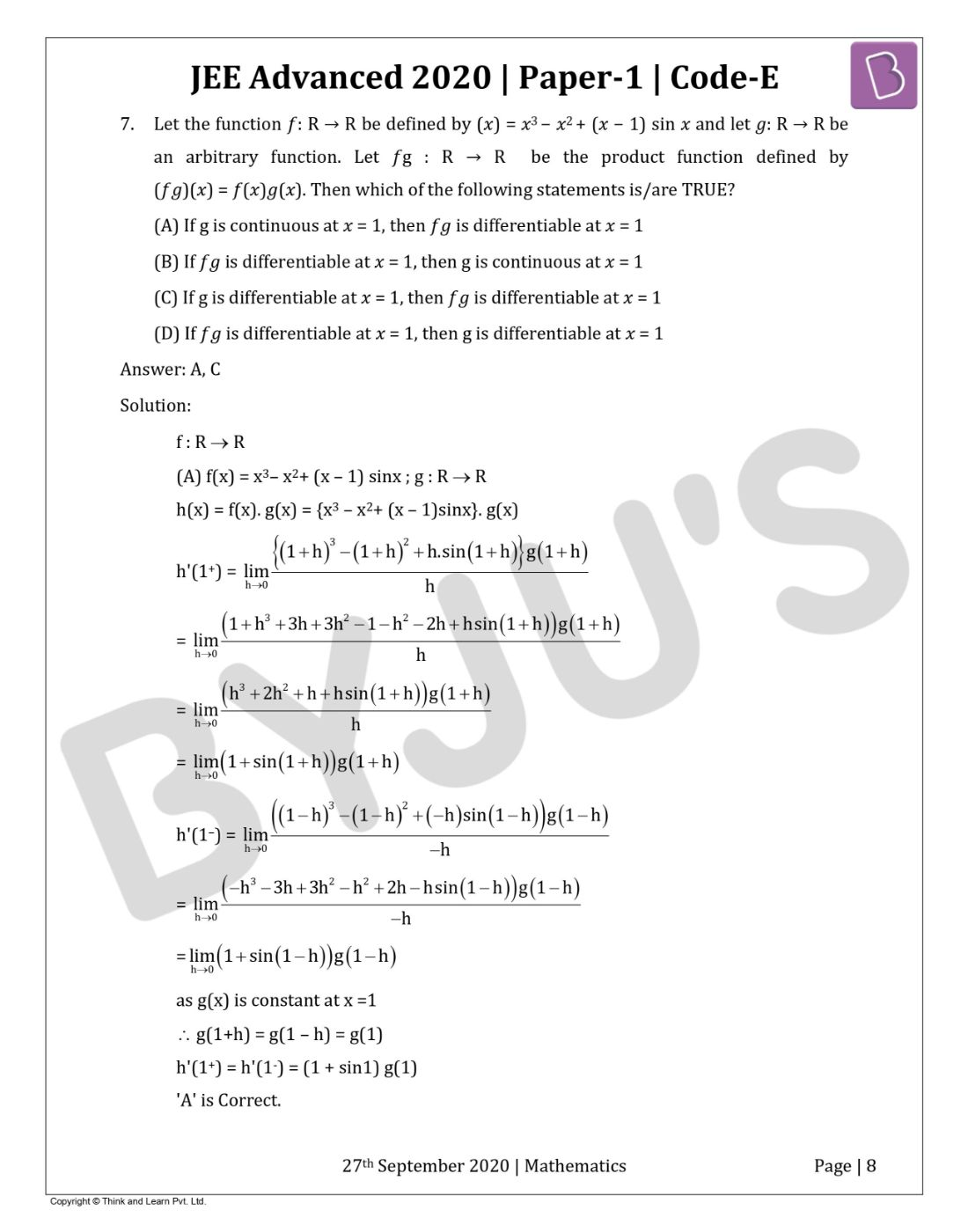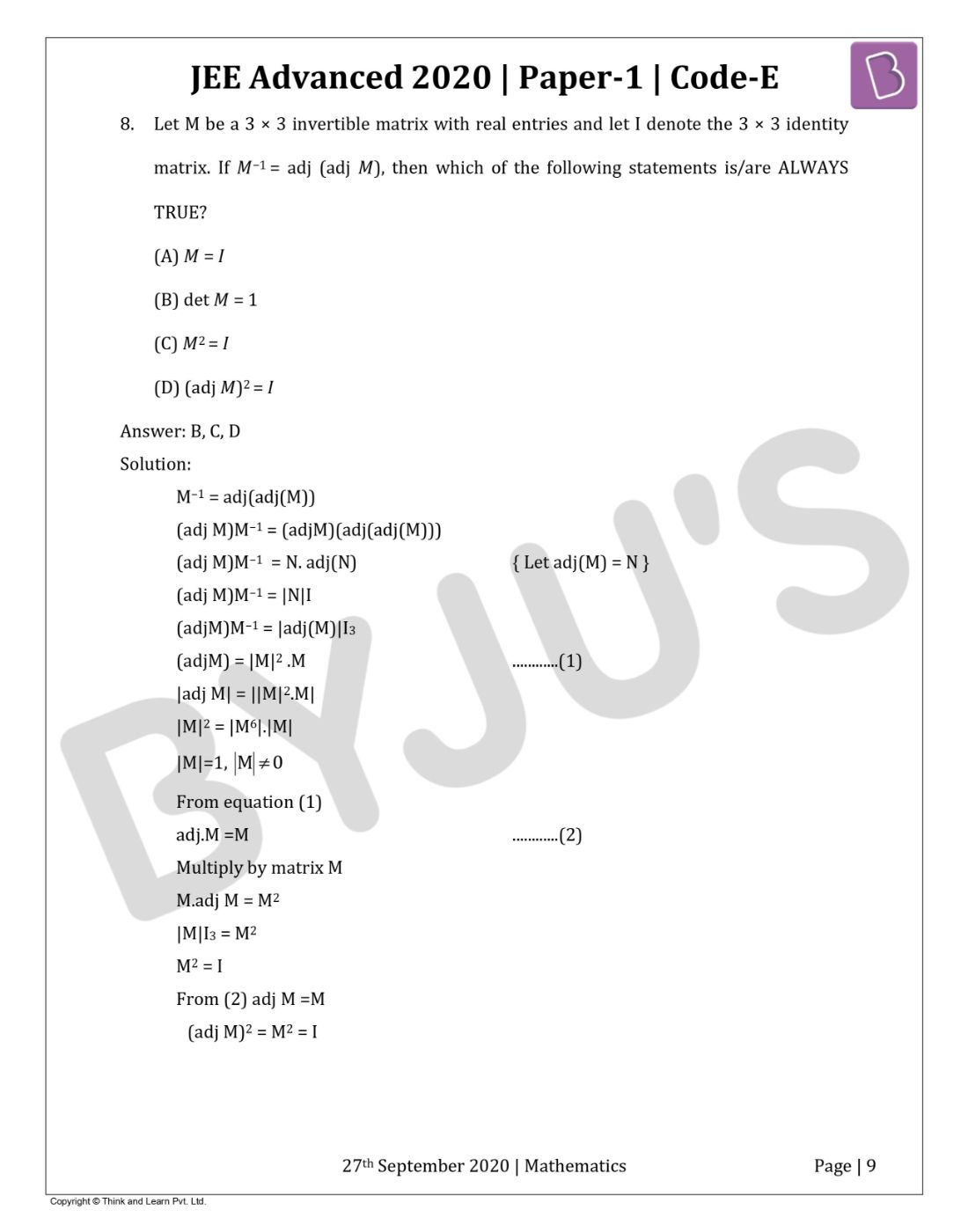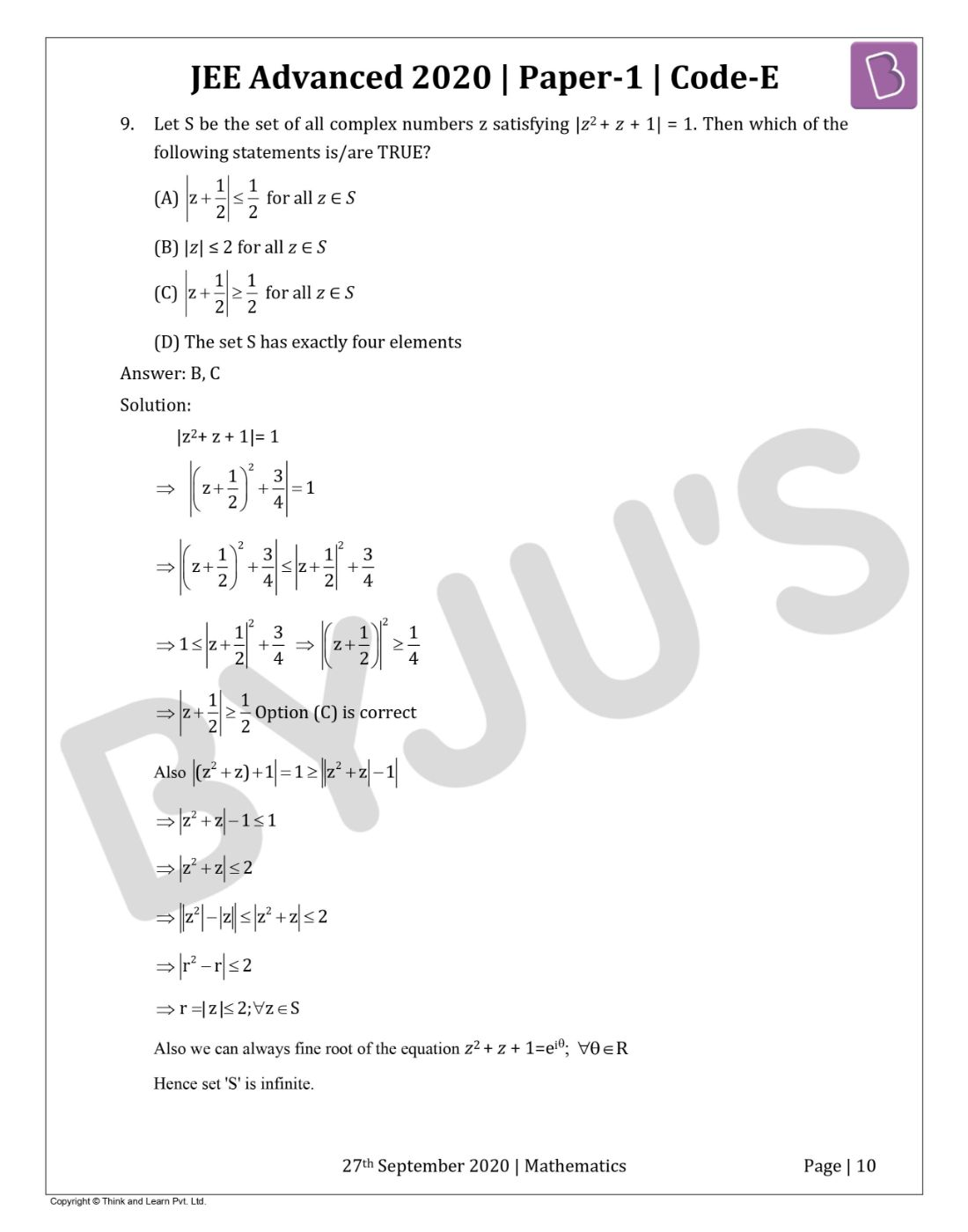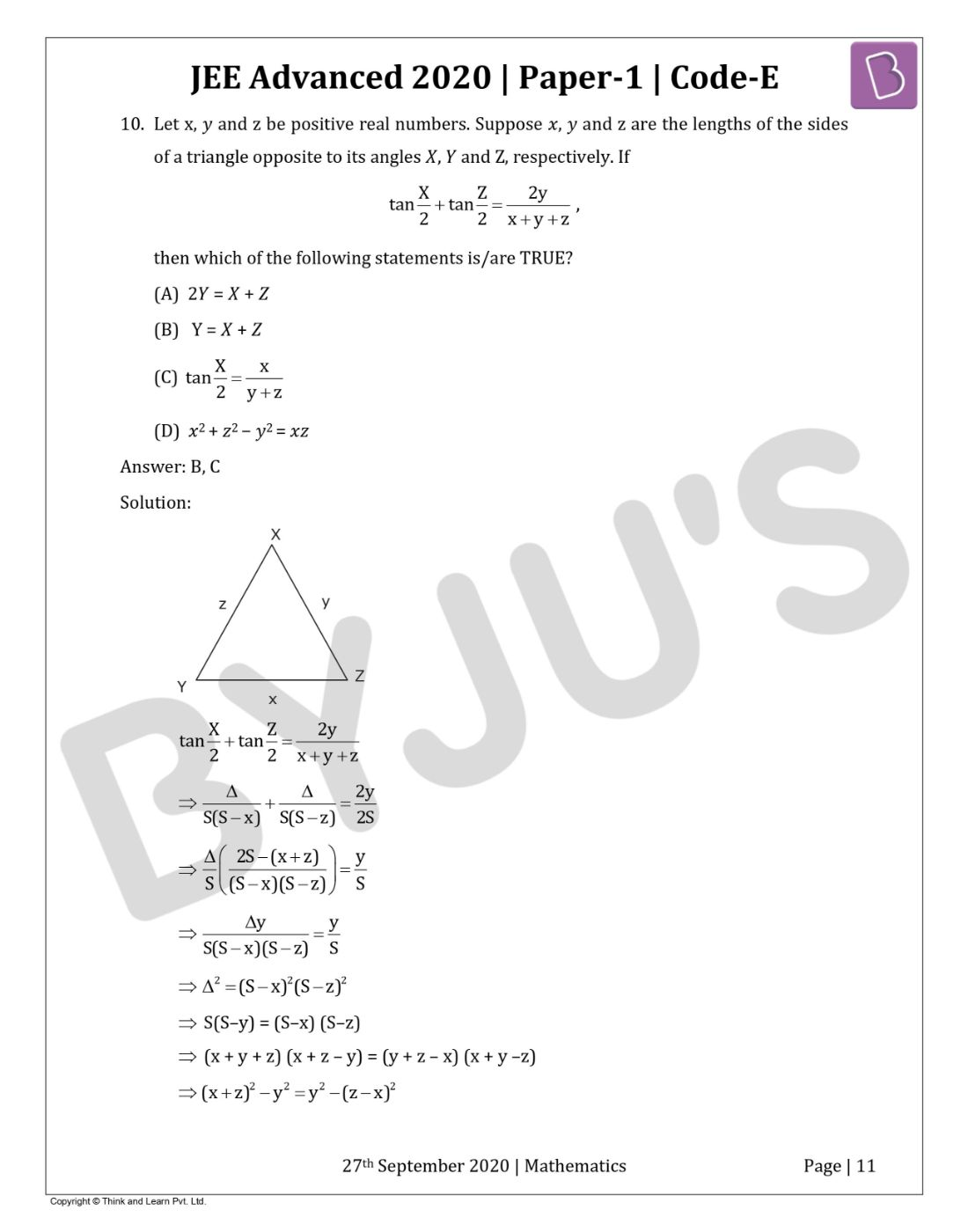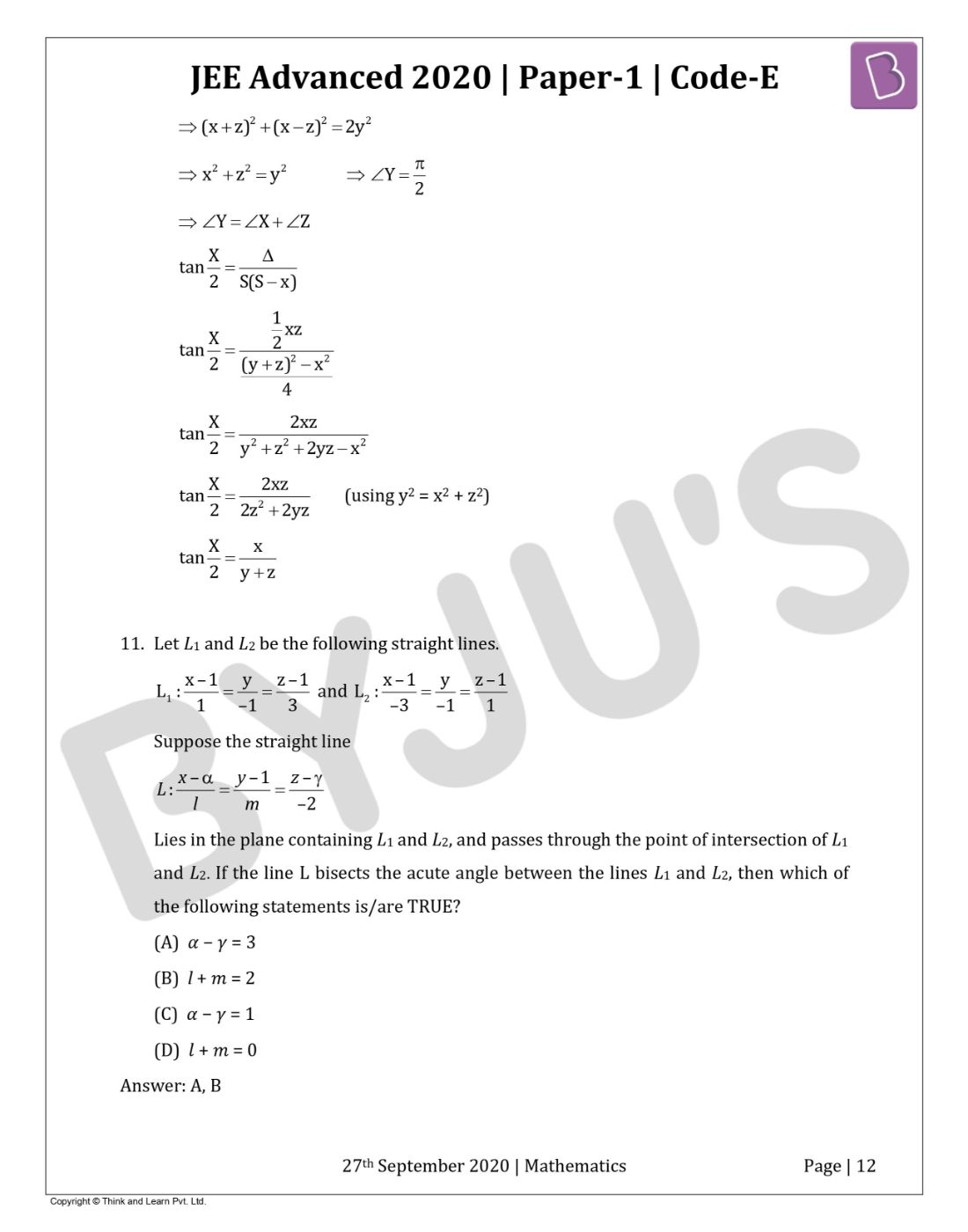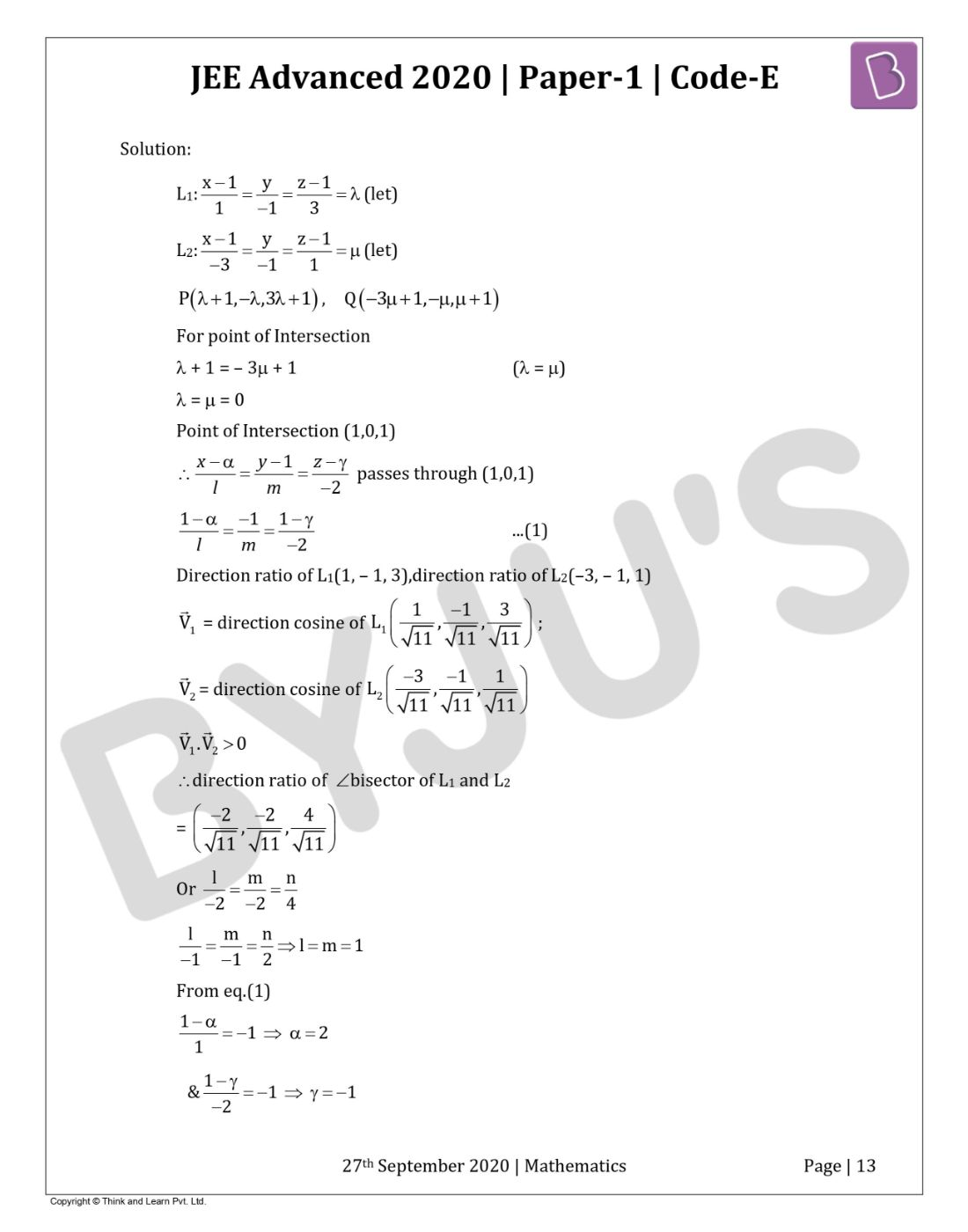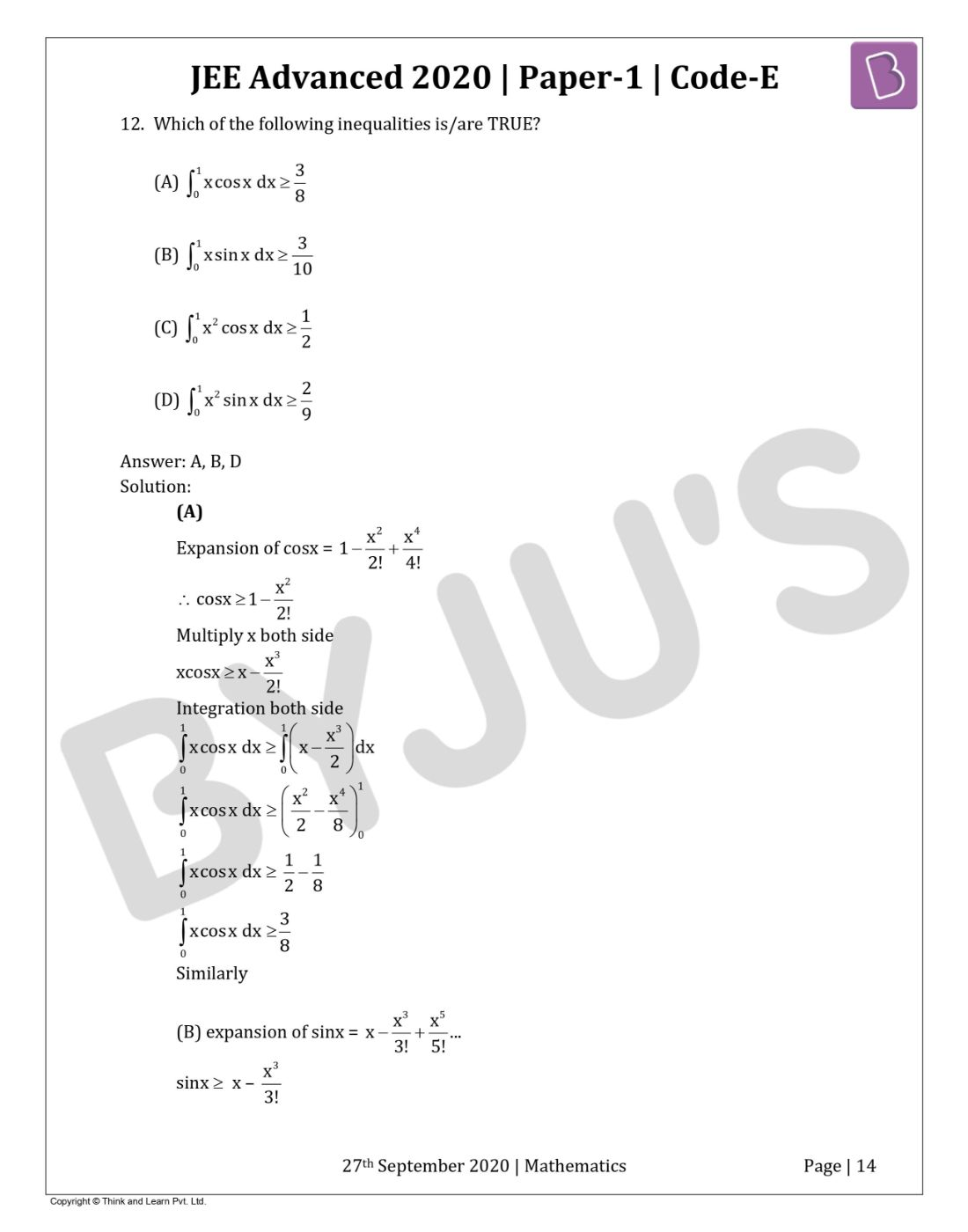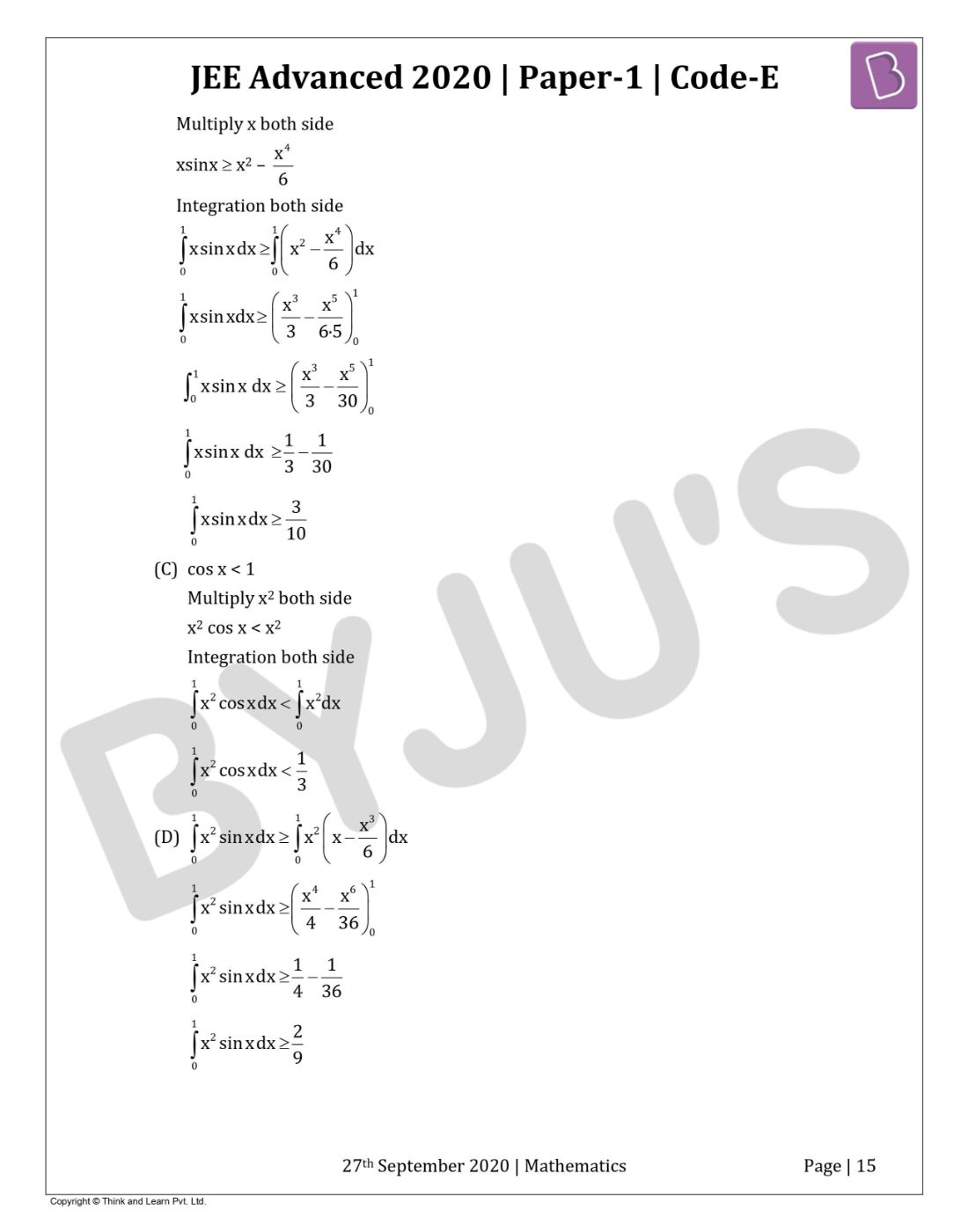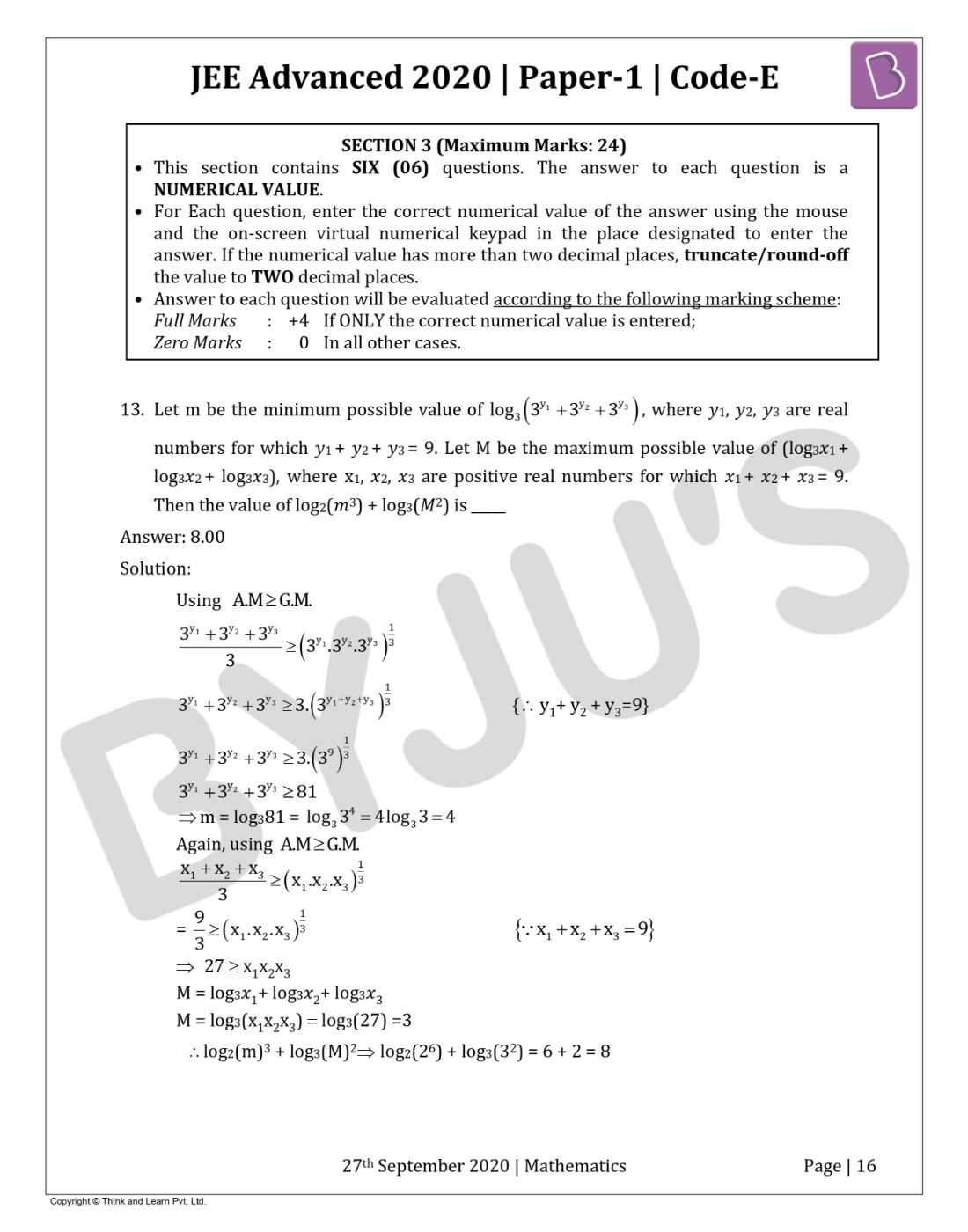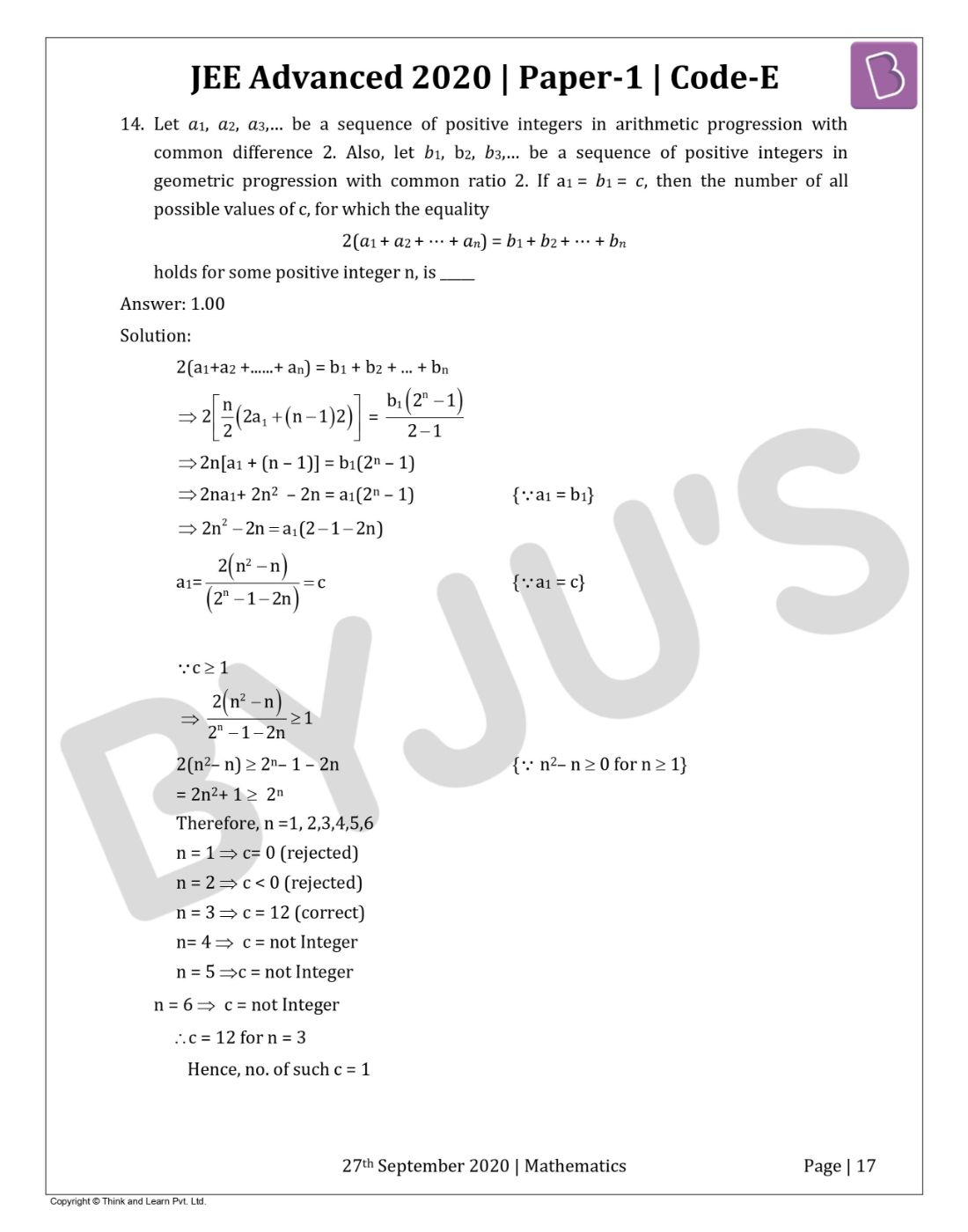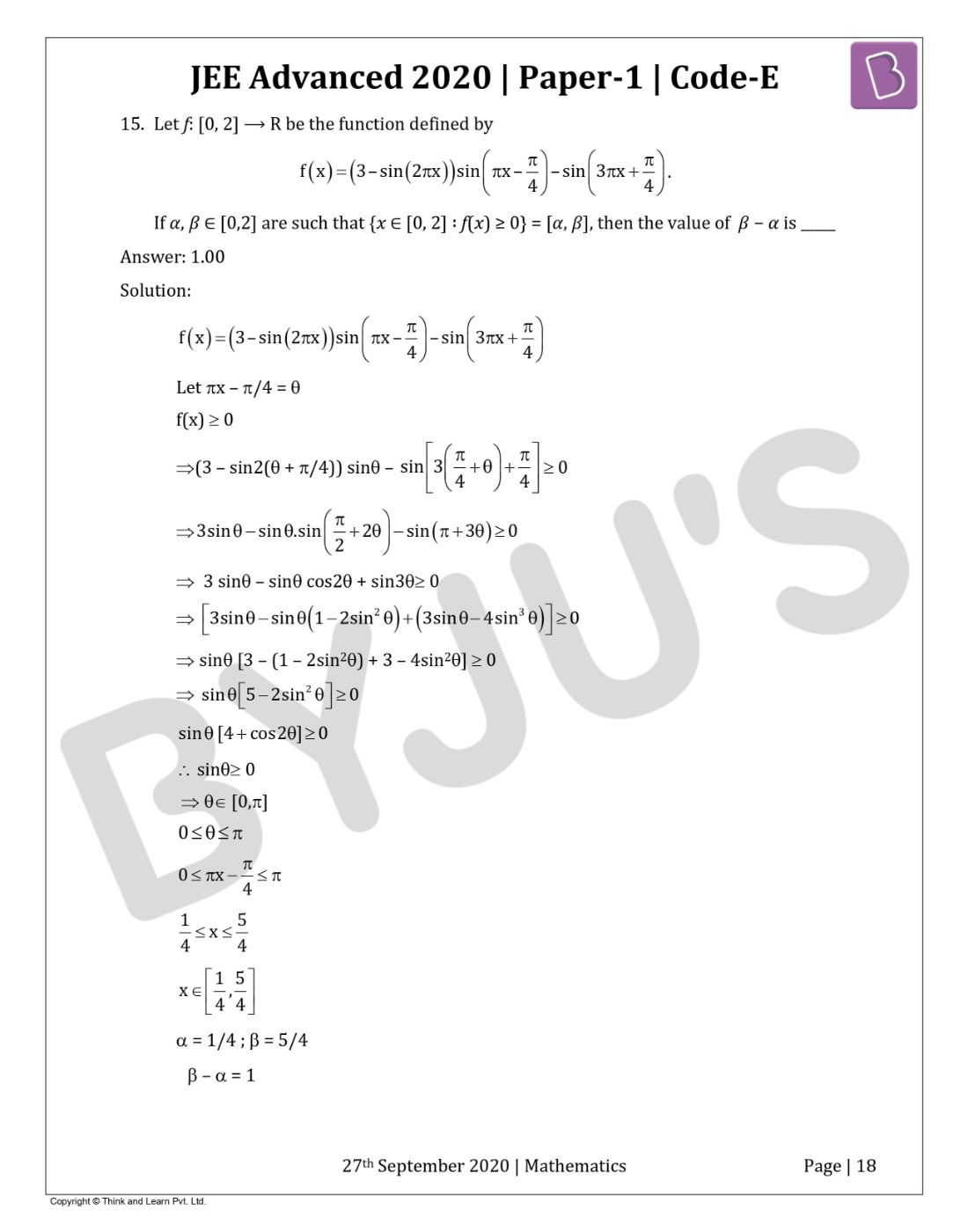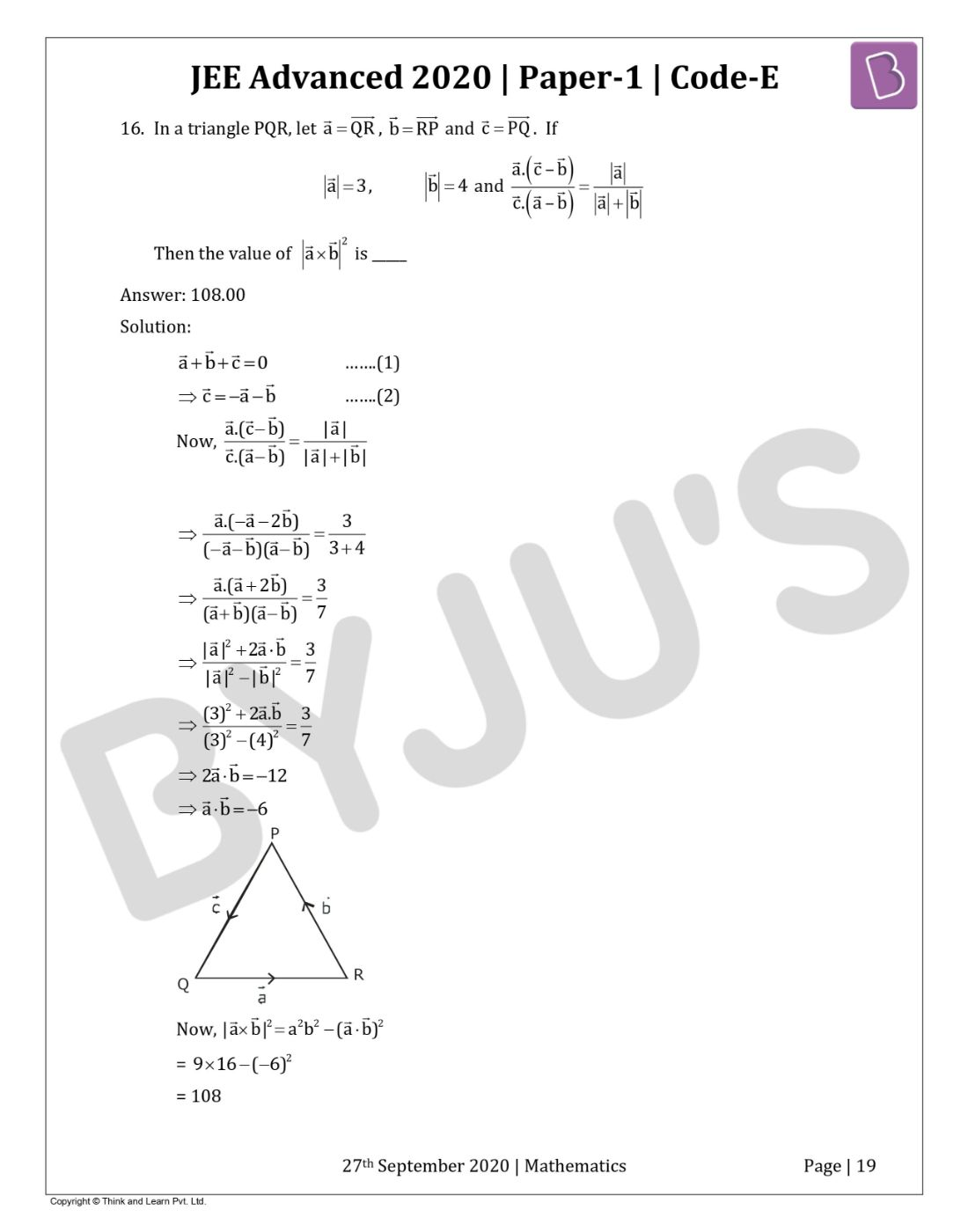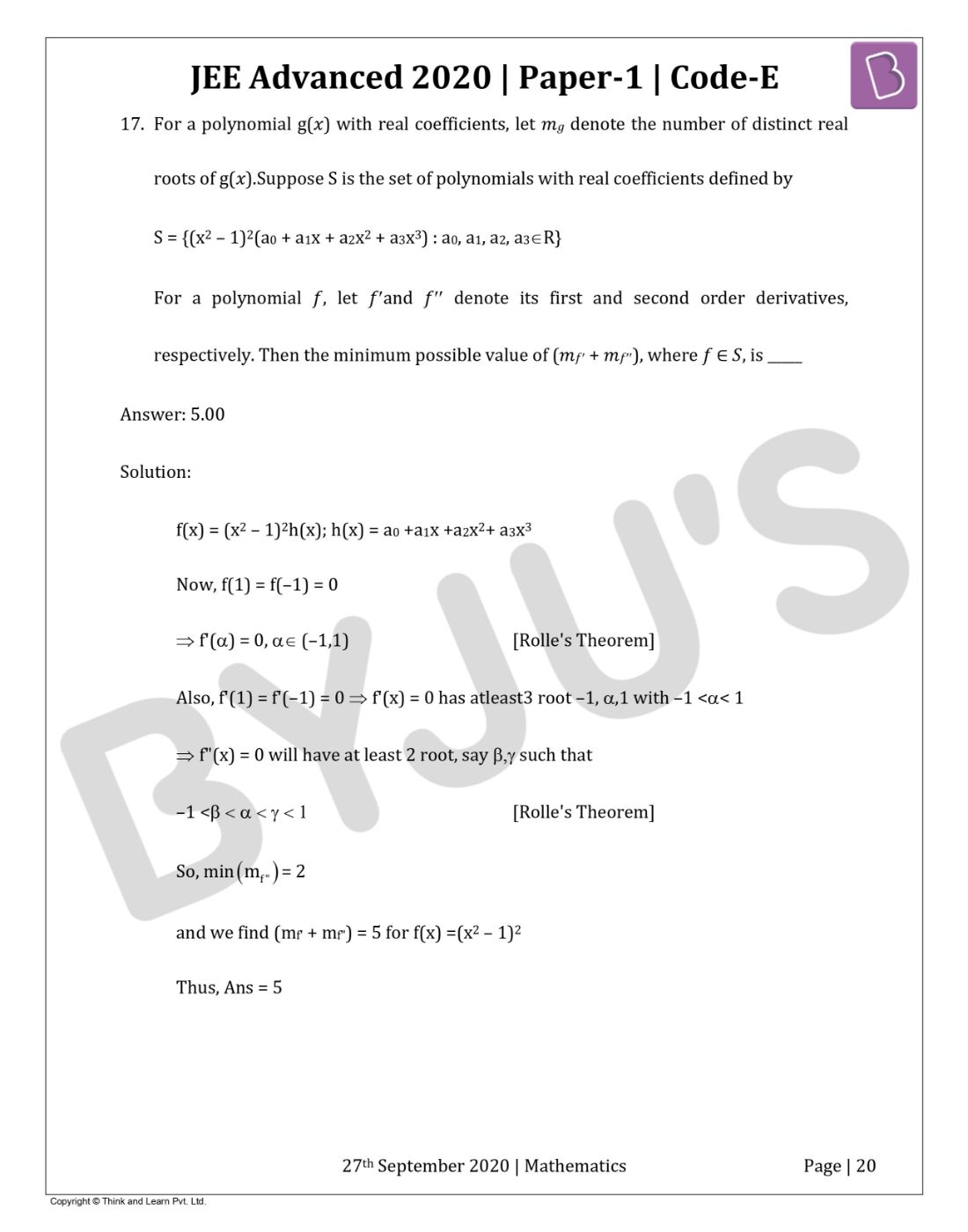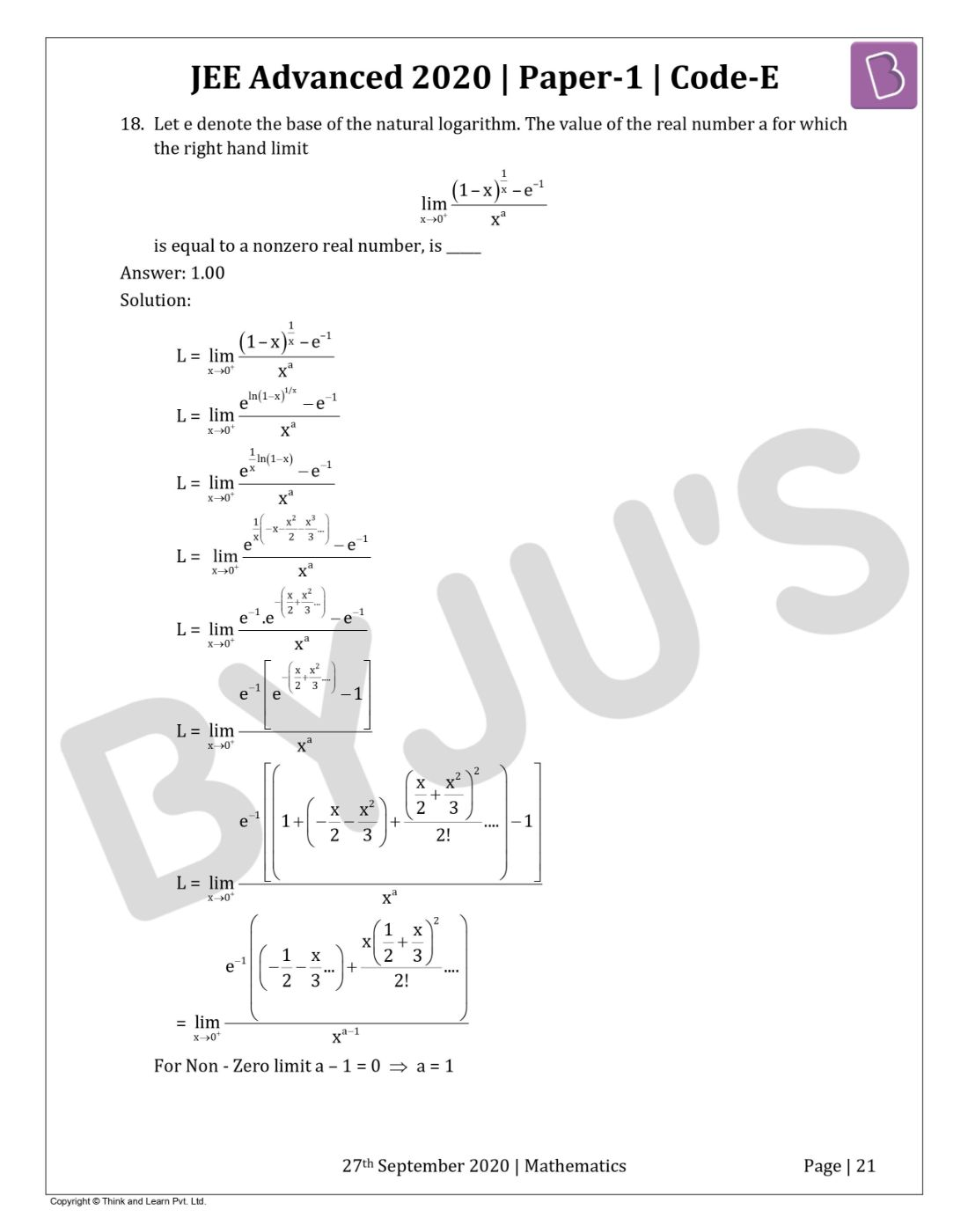Win up to 100% scholarship on Aakash BYJU'S JEE/NEET courses with ABNAT Win up to 100% scholarship on Aakash BYJU'S JEE/NEET courses with ABNAT

# JEE Advanced 2020 Maths Paper 1

Here students will get access to the complete set of questions from the Paper 1 Maths section of the JEE Advanced 2020 question paper. 18 questions were asked and a total of 66 marks were allocated for this section. There were MCQs, Numerical types question as well as paragraph based questions. The question paper provided here will help students get a better understanding of the question pattern, marking scheme and duration of the paper. Students can either view the paper directly or download it in a PDF format. They can use the question paper as a study guide and practice solving different problems. Detailed solutions are also provided to help the students find the right answer and study effectively for the entrance exam.
Paper 1 – Maths

Question 1. Suppose ??, ?? denote the distinct real roots of the quadratic polynomial ??2 + 20?? − 2020 and suppose ??, ?? denote the distinct complex roots of the quadratic polynomial

x2 − 20?? + 2020. Then the value of ???? (?? − ??) + ???? (?? − ??) + ???? (?? − ??) + ???? (?? − ??) is

a) 0
b) 8000
c) 8080
d) 16000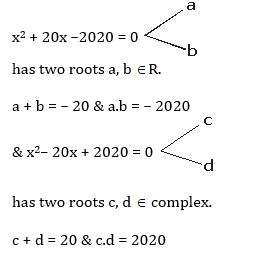Now

= ac (a – c) + ad (a – d) +bc (b – c) + bd (b – d)

= a2c – ac2 + a2d – ad2 +b2c – bc2+ b2d – bd2

= a2 (c +d) + b2 (c + d) – c2 (a + b) – d2 (a + b)

= (a2 + b2) (c + d) – (a + b) (c2 + d2)

= {(a+b)2 – 2ab} (c + d) – {(c + d)2 –2cd} (a + b)

Put value a, b, c and d then,

= {(-20)2 – 2(-2020)} (20) – {(20)2 – 2(2020)} (-20)}

= ((400 + 4040) (20) – (–20) ((20)2– 4040)

= 20[4440 – 3640]

20 = 16000

Question 2. If the function ??: R ? R is defined by (??) = |??| (??– sin??), then which of the following statements is TRUE?

a) ?? is one-one, but NOT onto
b) ?? is onto, but NOT one-one
c) ?? is BOTH one-one and onto
d) ?? is NEITHER one-one NOR onto

Given, f(x) = |x|(x – sinx)

f(–x) = –(|x|(x – sinx))

f(–x) = –f(x) ⇒ f(x) is odd, non-periodic and continuous function.

Now,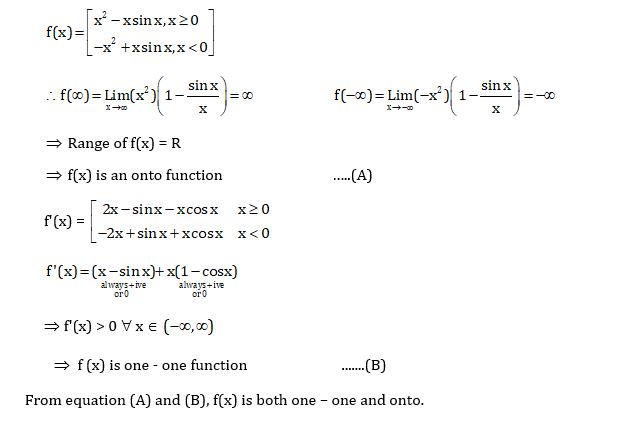Question 3. Let the functions: R ? R and g : R ? R be defined by

$$\begin{array}{l}f(x) = e^{x-1} – e^{-\left | x-1 \right |}\: and\: g(x) = \frac{1}{2}(e^{x-1} + e^{1-x})\end{array}$$
Then, the area of the region in the first quadrant bounded by the curves ?? = (??), ?? = g(??) and ?? = 0 is

a)

$$\begin{array}{l}(2-\sqrt{3})+\frac{1}{2}(e-e^{-1})\end{array}$$

b)
$$\begin{array}{l}(2+\sqrt{3})+\frac{1}{2}(e-e^{-1})\end{array}$$

c)
$$\begin{array}{l}(2-\sqrt{3})+\frac{1}{2}(e+e^{-1})\end{array}$$

d)
$$\begin{array}{l}(2+\sqrt{3})+\frac{1}{2}(e+e^{-1})\end{array}$$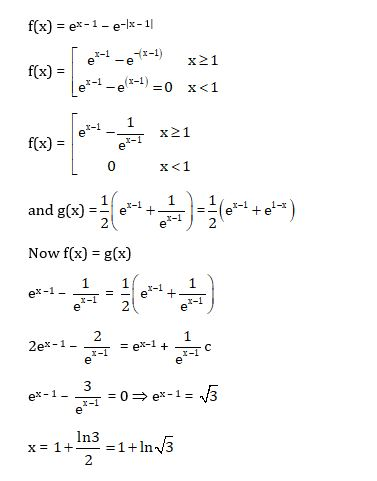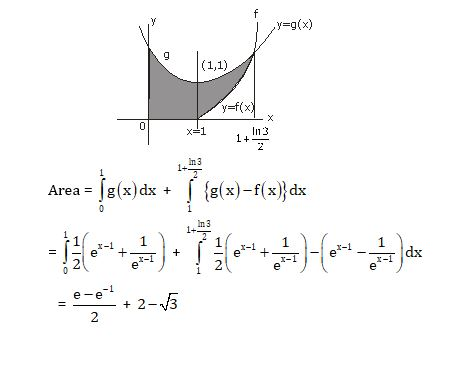Question 4. Let ??, ?? and λ be positive real numbers. Suppose P is an end point of the latus rectum of the parabola ??2 = 4λ??, and suppose the ellipse

$$\begin{array}{l}\frac{x^{2}}{a^{2}}+\frac{y^{2}}{b^{2}} = 1\end{array}$$
passes through the point??. If the tangents to the parabola and the ellipse at the point P are perpendicular to each other, then the eccentricity of the ellipse is

a) 1/√2
b) 1/2
c) 1/3
d) 2/5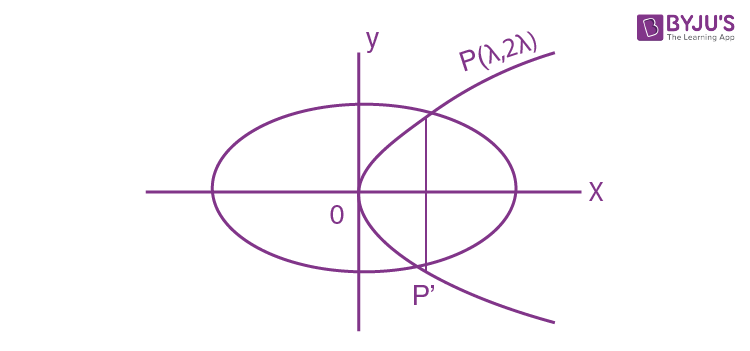P(λ, 2λ)

$$\begin{array}{l}y^{2} = 4\lambda x \Rightarrow \left ( \frac{dy}{dx} \right )_{A} = 1 = m_{1}\end{array}$$
…….(1)

Now E:

$$\begin{array}{l}\frac{x^{2}}{a^{2}}+\frac{y^{2}}{b^{2}} = 1\end{array}$$
Passes through P

$$\begin{array}{l}\frac{\lambda ^{2}}{a^{2}}+\frac{4\lambda ^{2}}{b^{2}} = 1 \Rightarrow \left ( \frac{dy}{dx} \right )_{A} = \frac{-b^{2}}{2a^{2}} = m_{2}\end{array}$$
$$\begin{array}{l}\frac{2\lambda }{2\lambda }X – \frac{\lambda }{a^{2}}\cdot \frac{b^{2}}{2\lambda } = -1\end{array}$$
…….(2)

From eq. (1) and (2)

m1. m2 = -1 ⇒ b2 = 2a2

$$\begin{array}{l}\frac{a^{2}}{b^{2}}=\frac{1}{2}\end{array}$$

for eccentricity of ellipse,

$$\begin{array}{l}e = \sqrt{1 – \frac{a^{2}}{b^{2}}} = \sqrt{1 – \frac{1}{2}} = \frac{1}{\sqrt{2}}\end{array}$$

Question 5. Let C1 and C2 be two biased coins such that the probabilities of getting head in a single toss are

$$\begin{array}{l}\frac{2}{3} and \frac{1}{3}\end{array}$$
, respectively. Suppose α is the number of heads that appear when C1 is tossed twice, independently, and suppose α is the number of heads that appear when C2 is tossed twice, independently. Then the probability that the roots of the quadratic polynomial ??2 − ???? + ?? are real and equal, is

a) 40/81
b) 20/81
c) 1/2
d) 1/4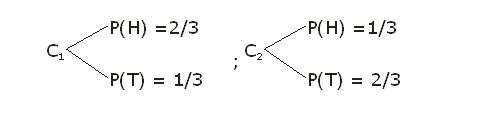Now roots of equation x2– αx + β = 0 are real and equal

∴ D= 0

α2 – 4β = 0

α2 = 4β

⇒ (α = 0, β = 0) or (α = 2, β = 1)

$$\begin{array}{l}P(E) = ^{2}C_{o}\left ( \frac{1}{3} \right )^{2}\cdot ^{2}C_{o}\left ( \frac{2}{3} \right )^{2} + ^{2}C_{2}\left ( \frac{2}{3} \right )^{2}\cdot ^{2}C_{1}\left ( \frac{1}{3} \right )\left ( \frac{2}{3} \right )\end{array}$$

$$\begin{array}{l}\frac{1}{9}\times\frac{4}{9}+\frac{4}{9}\times\frac{4}{9}=\frac{20}{81}\end{array}$$

Question 6. Consider all rectangles lying in the region

$$\begin{array}{l}\left \{ (x,y)\epsilon R\times R:0 \leq x\leq \frac{\pi }{2}\: and\: 0\leq y\leq 2sin(2x)\right \}\end{array}$$
and having one side on the x-axis. The area of the rectangle which has the maximum perimeter among all such rectangles, is

a)

$$\begin{array}{l}\frac{3\pi }{2}\end{array}$$

b) ??
c)
$$\begin{array}{l}\frac{\pi }{2\sqrt{3}}\end{array}$$

d)
$$\begin{array}{l}\frac{\pi\sqrt{3} }{2}\end{array}$$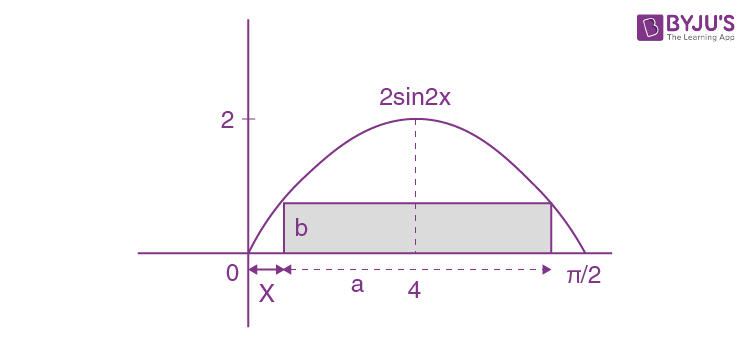Let sides of rectangle are a & b

Then, perimeter = 2a + 2b

p = 2(a + b)

Now, b = 2sin2x and b = 2sin(2x + 2a) ⇒ 2x + 2x + 2a = ??

$$\begin{array}{l}\left \{ x = \frac{\pi }{4}-\frac{a}{2}\right \}\end{array}$$

For perimeter maximum

P = 2a + 2b

P = ?? – 4x + 4sin2x

$$\begin{array}{l}\frac{dp}{dx} = -4+8cos2x = 8\left \{cos2x-\frac{1}{2} \right \}\end{array}$$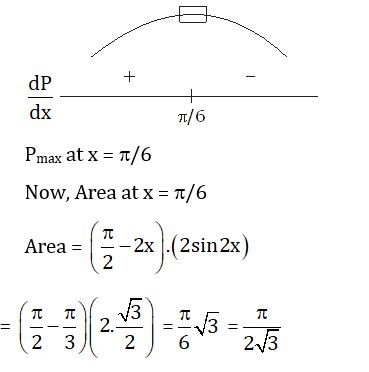Question 7. Let the function ??: R → R be defined by (??) = ??3 − ??2 + (?? − 1) sin ?? and let ??: R → R be an arbitrary function. Let ??g : R → R be the product function defined by (????)(??) = ??(??)??(??). Then which of the following statements is/are TRUE?

a) If g is continuous at ?? = 1, then ???? is differentiable at ?? = 1
b) If ???? is differentiable at ?? = 1, then g is continuous at ?? = 1
c) If g is differentiable at ?? = 1, then ???? is differentiable at ?? = 1
d) If ???? is differentiable at ?? = 1, then g is differentiable at ?? = 1

f : R → R

a) f(x) = x3– x2+ (x – 1) sinx ; g : R ? R

h(x) = f(x). g(x) = {x3 – x2+ (x – 1)sinx}. g(x)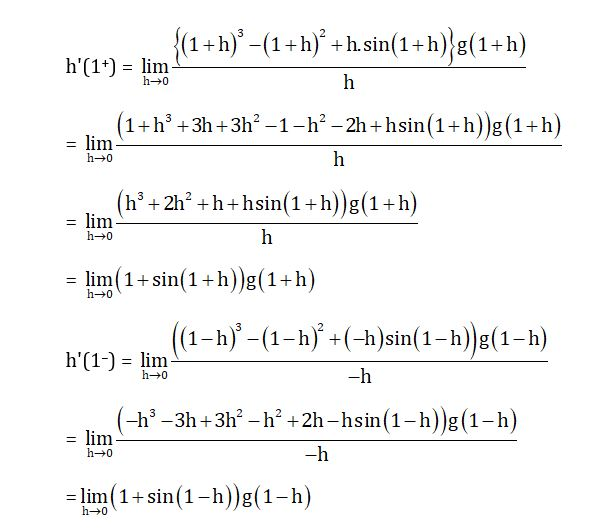as g(x) is constant at x =1

∴ g(1+h) = g(1 – h) = g(1)

h'(1+) = h'(1) = (1 + sin1) g(1)

‘a’ is correct.

Question 8. Let M be a 3 × 3 invertible matrix with real entries and let I denote the 3 × 3 identity matrix. If ??−1 = adj (adj ??), then which of the following statements is/are ALWAYS TRUE?

a) ?? = ??
b) det ?? = 1
c) ??2 = ??

|M|2 = |M6|.|M|

|M|=1, |M| ≠ 0

From equation (1)

Multiply by matrix M

|M|I3 = M2

M2 = I

(adj M)2 = M2 = I

Question 9. Let S be the set of all complex numbers z satisfying |??2 + ?? + 1| = 1. Then which of the following statements is/are TRUE?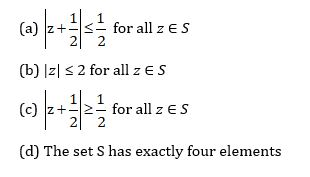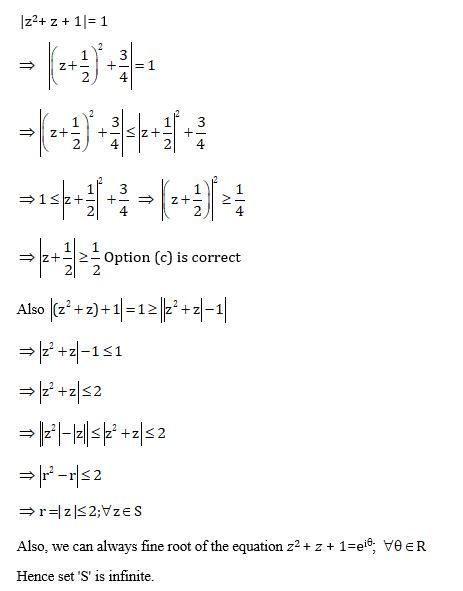Question 10. Let x, ?? and z be positive real numbers. Suppose ??, ?? and z are the lengths of the sides of a triangle opposite to its angles ??, ?? and Z, respectively. If

$$\begin{array}{l}tan\frac{X}{2}+tan\frac{Z}{2} =\frac{2y}{x+y+z}\end{array}$$
, then which of the following statements is/are TRUE?

a) 2?? = ?? + ??
b) Y = ?? + ??
c)

$$\begin{array}{l}tan\frac{X}{2} =\frac{x}{y+z}\end{array}$$

d) ??2 + ??2 − ??2 = ????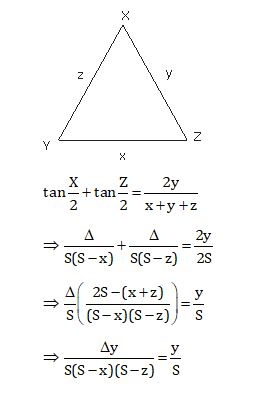?2 = (S – x)2(S – z)2

S(S–y) = (S–x) (S–z)

(x + y + z) (x + z – y) = (y + z – x) (x + y –z)

(x + z)2 – y2 = y2 – (z – x)2

(x + z)2 + (x – z)2 = 2y2

x 2 + z2 = y2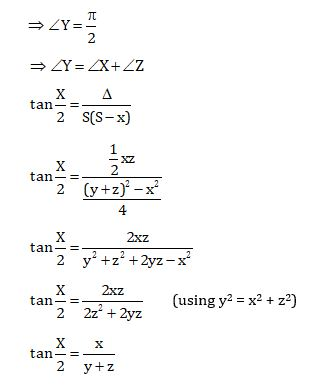Question 11. Let ??1 and ??2 be the following straight lines.

$$\begin{array}{l}L_{1}:\frac{x-1}{1} = \frac{y}{1} = \frac{z-1}{3}\end{array}$$
and
$$\begin{array}{l}L_{2}:\frac{x-1}{-3} = \frac{y}{-1} = \frac{z-1}{1}\end{array}$$

Suppose the straight line is

$$\begin{array}{l}L:\frac{x-\alpha }{l} = \frac{y-1}{m} = \frac{z-\gamma }{-2}\end{array}$$

Lies in the plane containing ??1 and ??2, and passes through the point of intersection of ??1 and

??2. If the line L bisects the acute angle between the lines ??1 and ??2, then which of the following statements is/are TRUE?

a) ?? − ?? = 3
b) l + ?? = 2
c) ?? − ?? = 1
d) ?? + ?? = 0

Solution: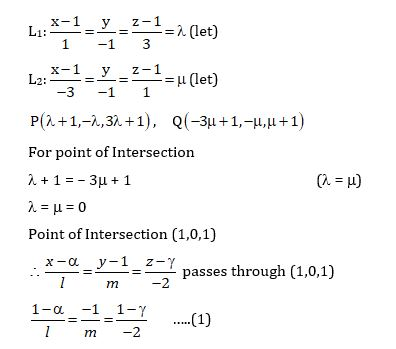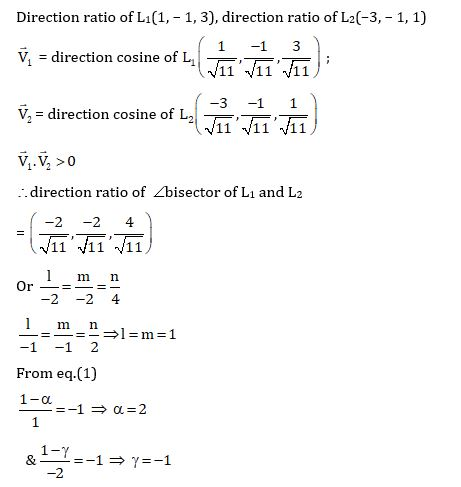Question 12. Which of the following inequalities is/are TRUE?

a)

$$\begin{array}{l}\int_{0}^{1}xcosx\: dx\geq \frac{3}{8}\end{array}$$

b)
$$\begin{array}{l}\int_{0}^{1}xsinx\: dx\geq \frac{3}{10}\end{array}$$

c)
$$\begin{array}{l}\int_{0}^{1}x^{2}cosx\: dx\geq \frac{1}{2}\end{array}$$

d)
$$\begin{array}{l}\int_{0}^{1}x^{2}sinx\: dx\geq \frac{2}{9}\end{array}$$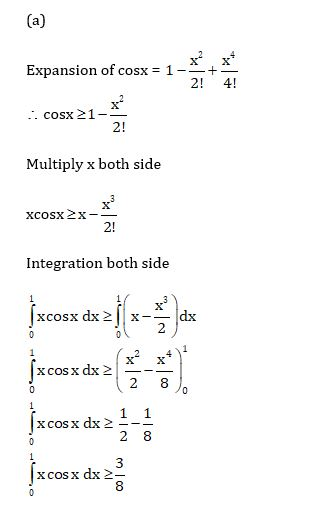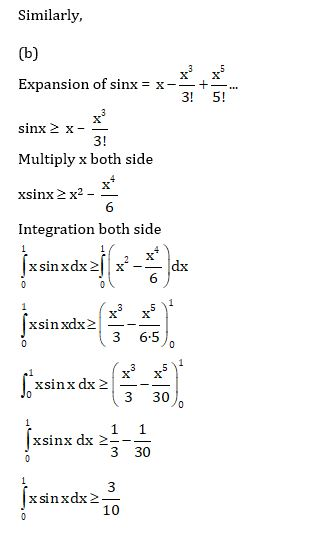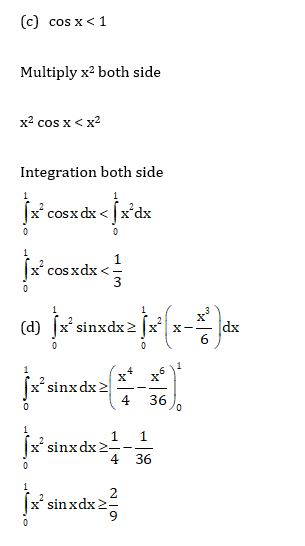Question 13. Let m be the minimum possible value of

$$\begin{array}{l}log_{3}(3^{y_{1}} + 3^{y_{2}} + 3^{y_{3}})\end{array}$$
, where ??1, ??2, ??3 are real numbers for which ??1 + ??2 + ??3 = 9. Let M be the maximum possible value of (log3??1 + log3??2 + log3??3), where x1, ??2, ??3 are positive real numbers for which ??1 + ??2 + ??3 = 9. Then the value of log2(??3) + log3(??2) is _____

Using AM = GM

$$\begin{array}{l}\frac{3^{y_{1}} + 3^{y_{2}} + 3^{y_{3}}}{3}\geq (3^{y_{1}}\cdot 3^{y_{2}}\cdot 3^{y_{3}})^{\frac{1}{3}}\end{array}$$
$$\begin{array}{l}{3^{y_{1}} + 3^{y_{2}} + 3^{y_{3}}}\geq 3\cdot (3^{y_{1}+y_{2}+y_{3}})^{\frac{1}{3}}\end{array}$$
{? y1+ y2 + y3=9}

$$\begin{array}{l}{3^{y_{1}} + 3^{y_{2}} + 3^{y_{3}}}\geq 3\cdot (3^{9})^{\frac{1}{3}}\end{array}$$
$$\begin{array}{l}{3^{y_{1}} + 3^{y_{2}} + 3^{y_{3}}}\geq 81\end{array}$$

m = log381 = log334 = 4log33 = 4

Again, using A.M ≥ G.M

$$\begin{array}{l}\frac{x_{1}+x_{2}+x_{3}}{3}\geq (x_{1}\cdot x_{2}\cdot x_{3})^{\frac{1}{3}}\end{array}$$

=

$$\begin{array}{l}\frac{9}{3}\geq (x_{1}\cdot x_{2}\cdot x_{3})^{\frac{1}{3}}\end{array}$$
{ ? x1+x2+x3 = 9}

⇒27 ≥ x1x2x3

M = log3??1+ log3??2+ log3??3

M = log3(x1x2x3) = log3(27) =3

∴ log2(m)3 + log3(M)2 ⇒ log2(26) + log3(32) = 6 + 2 = 8

Question 14. Let ??1, ??2, ??3,… be a sequence of positive integers in arithmetic progression with common difference 2. Also, let ??1, b2, ??3,… be a sequence of positive integers in geometric progression with common ratio 2. If a1 = ??1 = ??, then the number of all possible values of c, for which the equality 2(??1 + ??2 + ? + ????) = ??1 + ??2 + ? + ???? holds for some positive integer n, is _____

2(a1+a2 +……+ an) = b1 + b2 + … + bn

$$\begin{array}{l}2\left [ \frac{n}{2}(2a_{1} +(n-1)2) \right ] = \frac{b_{1}(2^{n}-1)}{2-1}\end{array}$$

⇒ 2n[a1 + (n – 1)] = b1(2n – 1)

⇒ 2na1+ 2n2 – 2n = a1(2n – 1) {? a1 = b1}

⇒ 2n2 – 2n = a1(2 – 1=2n)

$$\begin{array}{l}a_{1} = \frac{2(n^{2}-n)}{2^{n}-1-2n} = c\end{array}$$
{? a1 = c}

? c ≥ 1

$$\begin{array}{l}\Rightarrow \frac{2(n^{2}-n)}{2^{n}-1-2n}\geq 1\end{array}$$

2(n2– n) ≥ 2n– 1 – 2n {? n2– n ≥ 0 for n ≥ 1}

= 2n2+ 1 ≥ 2n

Therefore, n =1, 2,3,4,5,6

n = 1 ⇒ c= 0 (rejected)

n = 2 ⇒ c < 0 (rejected)

n = 3 ⇒ c = 12 (correct)

n = 4 ⇒ c = not Integer

n = 5 ⇒ c = not Integer

n = 6 ⇒ c = not Integer

? c = 12 for n = 3

Hence, no. of such c = 1

Question 15. Let f: [0, 2] ? R be the function defined by

$$\begin{array}{l}f(x) = (3-sin(2\pi x))sin\left (\pi x-\frac{\pi }{4} \right) – sin\left ( 3\pi x +\frac{\pi }{4} \right )\end{array}$$

If ??, ?? ∈ [0,2] are such that {?? ∈ [0, 2] : f(??) ≥ 0} = [??, ??], then the value of ?? − ?? is _____

$$\begin{array}{l}f(x) = (3-sin(2\pi x))sin\left (\pi x-\frac{\pi }{4} \right) – sin\left ( 3\pi x +\frac{\pi }{4} \right )\end{array}$$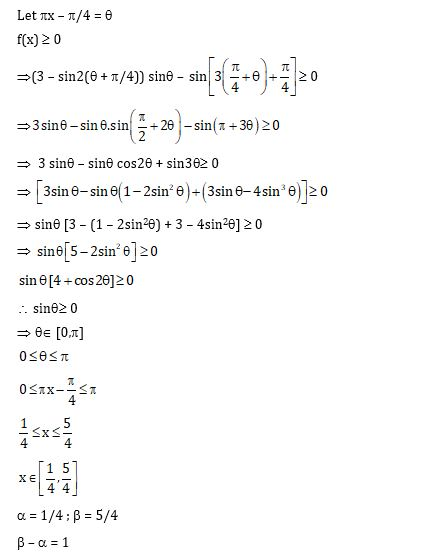Question 16. In a triangle PQR, let

$$\begin{array}{l}\vec{a} = \vec{QR}, \vec{b} = \vec{RP}\: and \: \vec{c} = \vec{PQ}\end{array}$$
. If

$$\begin{array}{l}|\vec{a}| = 3\end{array}$$
, and
$$\begin{array}{l}|\vec{b}| = 4\: and\: \frac{\vec{a}.(\vec{c} – \vec{b})}{\vec{c}.(\vec{a} – \vec{b})} = \frac{|\vec{a}|}{|\vec{a}|+|\vec{b}|}\end{array}$$

Then the value of is _____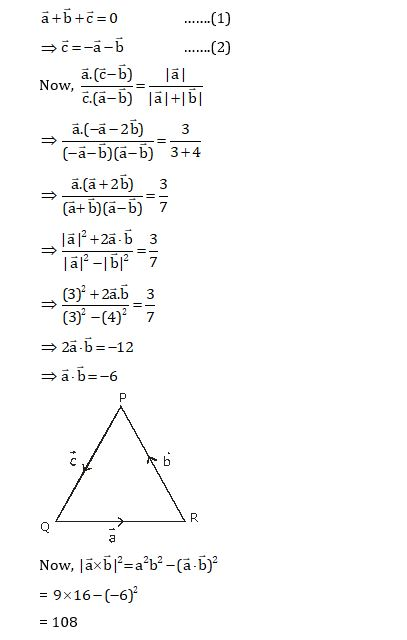Question 17. For a polynomial g(??) with real coefficients, let ???? denote the number of distinct real roots of g(??). Suppose S is the set of polynomials with real coefficients defined by S = {(x2 – 1)2(a0 + a1x + a2x2 + a3x3) : a0, a1, a2, a3∈R}

For a polynomial ??, let ??’and ??” denote its first and second order derivatives, respectively.

Then the minimum possible value of (????’ + ????”), where ?? ∈ ??, is _____

f(x) = (x2 – 1)2h(x); h(x) = a0 +a1x +a2x2+ a3x3

Now, f(1) = f(–1) = 0

⇒ f'(α) = 0, α∈ (–1,1) [Rolle’s Theorem]

Also, f'(1) = f'(–1) = 0 ⇒ f'(x) = 0 has at least 3 root –1, α,1 with –1 <α< 1

⇒ f”(x) = 0 will have at least 2 roots, say β, γ such that

–1 < β < α < γ < 1 [Rolle’s Theorem]

So, min= 2

and we find (mf’ + mf”) = 5 for f(x) =(x2 – 1)2

Thus, Ans = 5

Question 18. Let e denote the base of the natural logarithm. The value of the real number a for which the right-hand limit is equal to a nonzero real number, is _____